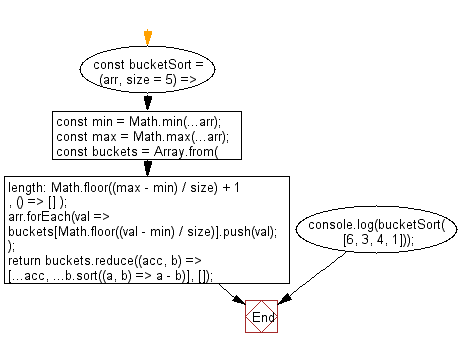﻿ PHP: Sort a list of elements using Strand sort - w3resource

# PHP Searching and Sorting Algorithm: Strand sort

## PHP Searching and Sorting Algorithm: Exercise-15 with Solution

Write a PHP program to sort a list of elements using Strand sort.

This is a way of sorting numbers by extracting shorter sequences of already sorted numbers from an unsorted list.

Sample Solution :

PHP Code :

``````<?php
foreach (array(100, 0, 2, 5, -1, 4, 1) as \$v)
\$lst->push(\$v);
foreach (strandSort(\$lst) as \$v)
echo "\$v ";
echo " ".PHP_EOL;
while (!\$lst->isEmpty()) {
\$sorted->push(\$lst->shift());
foreach (\$lst as \$item) {
if (\$sorted->top() <= \$item) {
\$sorted->push(\$item);
} else {
\$remain->push(\$item);
}
}
\$result = _merge(\$sorted, \$result);
\$lst = \$remain;
}
return \$result;
}
while (!\$left->isEmpty() && !\$right->isEmpty()) {
if (\$left->bottom() <= \$right->bottom()) {
\$res->push(\$left->shift());
} else {
\$res->push(\$right->shift());
}
}
foreach (\$left as \$v)  \$res->push(\$v);
foreach (\$right as \$v) \$res->push(\$v);
return \$res;
}
?>
```
```

Sample Output:

```-1 0 1 2 4 5 100
```

Flowchart :PHP Code Editor:

Have another way to solve this solution? Contribute your code (and comments) through Disqus.

What is the difficulty level of this exercise?

Test your Programming skills with w3resource's quiz.

﻿